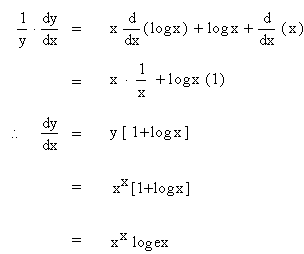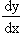Home MonkeyNotes Printable Notes Digital Library Study Guides Study Smart Parents Tips College Planning Test Prep Fun Zone Help / FAQ How to Cite New Title Request

4.13 Logarithmic Differentiation

In order to find the derivative of

1. a function which is the product or quotient of a number of functions

or

2. a function of the form the [ f ( x ) ]g ( x ) where f ( x ) and g ( x ) are both derivable, it is usually advisable to take logarithm of the function first and then differentiate. The process is known as the logarithmic differentiation.

Example 61

If y = xx Find y

Solution :    y  =  xx

Taking logarithms of both sides, we have

log y   =   log xx    =    x log x

Differentiating w. r. to x, we getExample 62    If   y =  ( log x ) log x FindYour browser does not support the IFRAME tag.

Solution:    y  =  ( log x ) log x then we have

log y = log ( log x ) log x

= log x . log ( log x )Index

4. 1 Derivability At A Point
4. 2 Derivability In An Interval
4. 3 Derivability And Continuity Of A Function At A Point
4. 4 Some Counter Examples

4. 5 Interpretation Of Derivatives
4. 6 Theorems On Derivatives (differentiation Rules)
4. 7 Derivatives Of Standard Functions
4. 8 Derivative Of A Composite Function
4. 9 Differentiation Of Implicit Functions
4.10 Derivative Of An Inverse Function
4.11 Derivatives Of Inverse Trigonometric Functions
4.12 Derivatives Of Exponential & Logarithmic Functions
4.13 Logarithmic Differentiation
4.14 Derivatives Of Functions In Parametric Form
4.15 Higher order Derivatives

Chapter 5